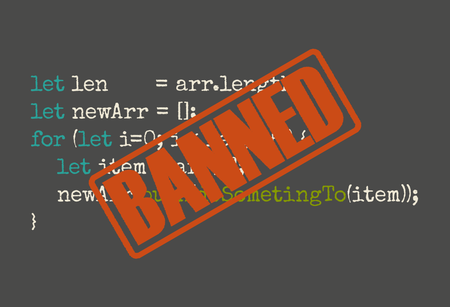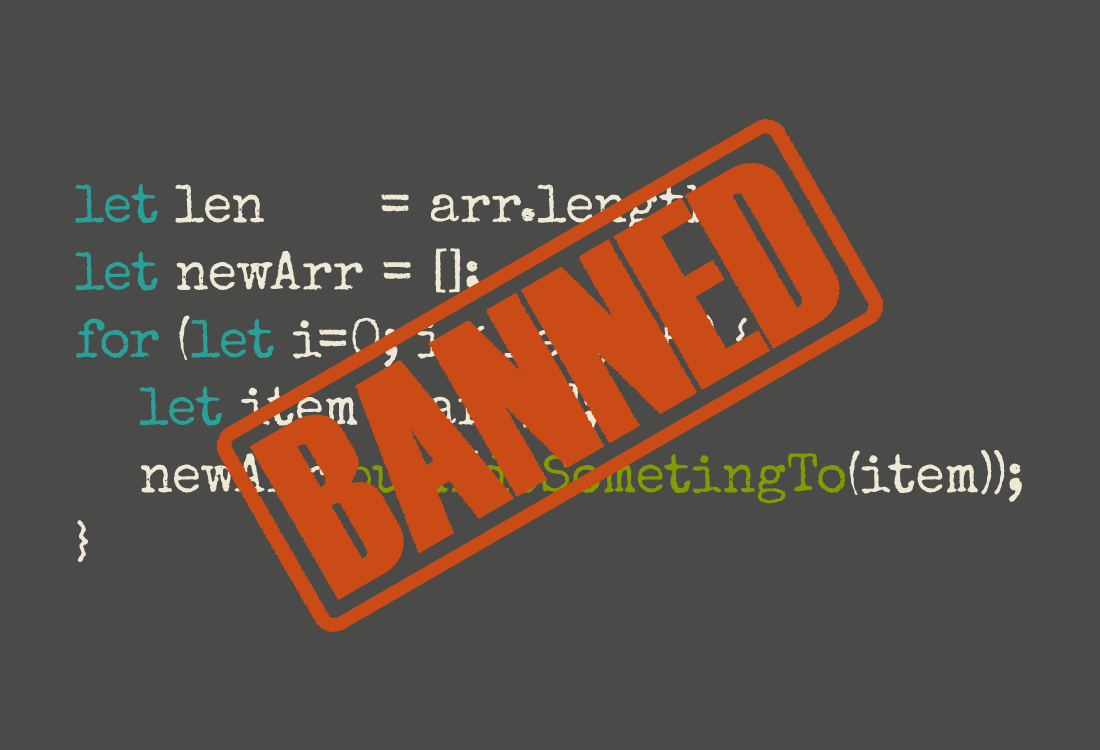Doraemonls## 不使用循环的JavaScript“循环是一种命令式的控制结构，它不利于重用，也无法方便的和其他操作结合。除此之外，它还意味着代码在不断改变或者不断响应在新迭代中的变化。” —Luis Atencio

## Loops

We’ve been saying that control structures like loops introduce complexity. But so far we’ve not seen any evidence of how that happens. So let’s take a look at how loops in JavaScript work. 上面我们已经讲过类似循环的控制结构会引入复杂性问题。不过还没有明确的证据解释这一切如何发生。所以我们不妨来看看JavaScript的循环是如何工作的。

JavaScript至少有四或五种循环方式。最基础的是while循环。不过，我们首先要创建一个可以循环的数组。

``````// oodlify :: String -> String
function oodlify(s) {
return s.replace(/[aeiou]/g, 'oodle');
}

const input = [
'John',
'Paul',
'George',
'Ringo',
];
``````

``````let i = 0;
const len = input.length;
let output = [];
while (i < len) {
let item = input[i];
let newItem = oodlify(item);
output.push(newItem);
i = i + 1;
}

``````

``````const len = input.length;
let output = [];
for (let i = 0; i < len; i = i + 1) {
let item = input[i];
let newItem = oodlify(item);
output.push(newItem);
}

``````

``````let output = [];
for (let item of input) {
let newItem = oodlify(item);
output.push(newItem);
}

``````

## Mapping

for…of循环比for-loop更简洁，但我们还需要一些例子。让我们初始化一个`output`数组，并在循环内对调用`push()` 方法。我们可以让代码更加简洁明了，要怎么做？让我们把这个例子扩展一点。

``````const fellowship = [
'frodo',
'sam',
'gandalf',
'aragorn',
'boromir',
'legolas',
'gimli',
];

const band = [
'John',
'Paul',
'George',
'Ringo',
];

``````

``````let bandoodle = [];
for (let item of band) {
let newItem = oodlify(item);
bandoodle.push(newItem);
}

let floodleship = [];
for (let item of fellowship) {
let newItem = oodlify(item);
floodleship.push(newItem);
}

``````

This works. And code that works is better than code that doesn’t. But, it’s repetitive—not very DRY. We can refactor it to reduce some of the repetition. So, we create a function: 恩，这段代码行得通。行得通的代码起码比无效的代码好。不过，它有重复，所以不符合DRY的原则。为了避免重复，让我们重构一下代码。那我们新建一个方法：

``````function oodlifyArray(input) {
let output = [];
for (let item of input) {
let newItem = oodlify(item);
output.push(newItem);
}
return output;
}

let bandoodle = oodlifyArray(band);
let floodleship = oodlifyArray(fellowship);

``````

``````function izzlify(s) {
return s.replace(/[aeiou]+/g, 'izzle');
}

``````

`oodlifyArray()`就失效了。不过可以新建类似的`izzlifyArray()` ，重复以上的工作。这样我们就可以对比一下：

``````function oodlifyArray(input) {
let output = [];
for (let item of input) {
let newItem = oodlify(item);
output.push(newItem);
}
return output;
}

function izzlifyArray(input) {
let output = [];
for (let item of input) {
let newItem = izzlify(item);
output.push(newItem);
}
return output;
}

``````

``````function map(f, a) {
let output = [];
for (let item of a) {
output.push(f(item));
}
return output;
}

``````

``````function map(f, a) {
if (a.length === 0) { return []; }
return [f(a)].concat(map(f, a.slice(1)));
}

``````

``````let bandoodle     = band.map(oodlify);
let floodleship   = fellowship.map(oodlify);
let bandizzle     = band.map(izzlify);
let fellowshizzle = fellowship.map(izzlify);

``````

Note the lack of indenting. Note the lack of loops. Sure, there might be a loop going on somewhere, but that’s not our concern any more. This code is now both concise and expressive. It is also simple. 请注意，这里没有缩进，没有循环。当然了，肯定在某个地方有个循环，但我们不需要了解。这段代码清晰明了，也容易理解。

## Reducing

Now, `map` is very handy, but it doesn’t cover every kind of loop we might need. It’s only useful if you want to create an array of exactly the same length as the input. But what if we wanted to add up an array of numbers? Or find the shortest string in a list? Sometimes we want to process an array and reduce it down to just one value. 现在你知道`map`很容易上手，但它也并不能满足可能遇到的各种循环情况。只有在你需要创建一个长度一样的数组时，这个方法很有效。但如果需要求数组所有值的和呢？或者找到其中最短的字符串呢？有些时候我们就需要对数组进行Reduce操作，把它输出的更小，直到只有一个值为止。

``````const heroes = [
{name: 'Hulk', strength: 90000},
{name: 'Spider-Man', strength: 25000},
{name: 'Hawk Eye', strength: 136},
{name: 'Thor', strength: 100000},
{name: 'Black Widow', strength: 136},
{name: 'Vision', strength: 5000},
{name: 'Scarlet Witch', strength: 60},
{name: 'Mystique', strength: 120},
{name: 'Namora', strength: 75000},
];

``````

``````let strongest = {strength: 0};
for (let hero of heroes) {
if (hero.strength > strongest.strength) {
strongest = hero;
}
}

``````

``````let combinedStrength = 0;
for (let hero of heroes) {
combinedStrength += hero.strength;
}

``````

``````function greaterStrength(champion, contender) {
return (contender.strength > champion.strength) ? contender : champion;
}

return tally + hero.strength;
}

const initialStrongest = {strength: 0};
let working = initialStrongest;
for (hero of heroes) {
working = greaterStrength(working, hero);
}
const strongest = working;

const initialCombinedStrength = 0;
working = initialCombinedStrength;
for (hero of heroes) {
}
const combinedStrength = working;

``````

``````function reduce(f, initialVal, a) {
let working = initialVal;
for (let item of a) {
working = f(working, item);
}
return working;
}

``````

``````const strongestHero = heroes.reduce(greaterStrength, {strength: 0});

``````

## Filtering

``````const heroes = [
{name: 'Hulk', strength: 90000, sex: 'm'},
{name: 'Spider-Man', strength: 25000, sex: 'm'},
{name: 'Hawk Eye', strength: 136, sex: 'm'},
{name: 'Thor', strength: 100000, sex: 'm'},
{name: 'Black Widow', strength: 136, sex: 'f'},
{name: 'Vision', strength: 5000, sex: 'm'},
{name: 'Scarlet Witch', strength: 60, sex: 'f'},
{name: 'Mystique', strength: 120, sex: 'f'},
{name: 'Namora', strength: 75000, sex: 'f'},
];

``````

1. 找到所有的女性超级英雄；

2. 找到所有力量值大于500的英雄。

``````let femaleHeroes = [];
for (let hero of heroes) {
if (hero.sex === 'f') {
femaleHeroes.push(hero);
}
}

let superhumans = [];
for (let hero of heroes) {
if (hero.strength >= 500) {
superhumans.push(hero);
}
}

``````

``````function isFemaleHero(hero) {
return (hero.sex === 'f');
}

function isSuperhuman(hero) {
return (hero.strength >= 500);
}

let femaleHeroes = [];
for (let hero of heroes) {
if (isFemaleHero(hero)) {
femaleHeroes.push(hero);
}
}

let superhumans = [];
for (let hero of heroes) {
if (isSuperhuman(hero)) {
superhumans.push(hero);
}
}

``````

``````function filter(predicate, arr) {
let working = [];
for (let item of arr) {
if (predicate(item)) {
working = working.concat(item);
}
}
}

const femaleHeroes = filter(isFemaleHero, heroes);
const superhumans  = filter(isSuperhuman, heroes);

``````

``````const femaleHeroes = heroes.filter(isFemaleHero);
const superhumans  = heroes.filter(isSuperhuman);

``````

## Finding

``````function isBlackWidow(hero) {
return (hero.name === 'Black Widow');
}

const blackWidow = heroes.filter(isBlackWidow);

``````

``````function find(predicate, arr) {
for (let item of arr) {
if (predicate(item)) {
return item;
}
}
}

const blackWidow = find(isBlackWidow, heroes);

``````

JavaScript也提供了这个函数，所以我们也不需要再写一次。

```````const blackWidow = heroes.find(isBlackWidow);`

``````

## 总结

1. 消除循环结构，让代码更加简洁易读。

2. 确定问题模式，找到对应的内置方法，即`map`, `reduce`, `filter`, 或者`find`其中的一种。

3. 把对整个数组的处理逻辑简化成对每个数据的处理。

## 2月23号的一点更新

``````function processStrength({strongestHero, combinedStrength}, hero) {
return {
strongestHero: greaterStrength(strongestHero, hero),